# Ciscn 2022 Re&可信计算 WriteUp

Posted by Qfrost on 2022-05-30
Estimated Reading Time 16 Minutes
Words 3.4k In Total
Viewed Times

## Reverse

### baby_tree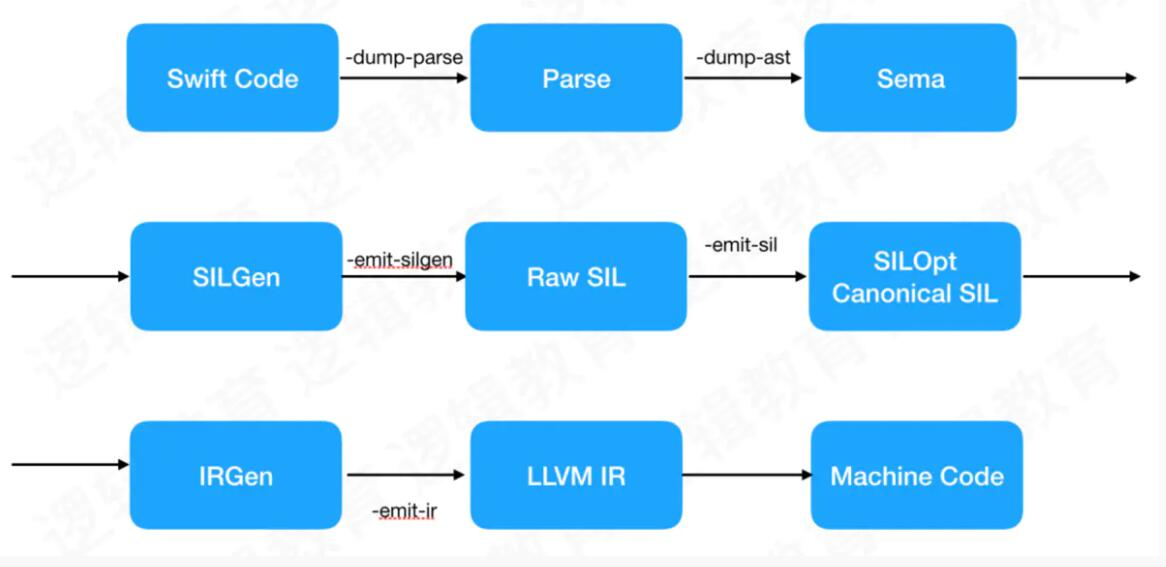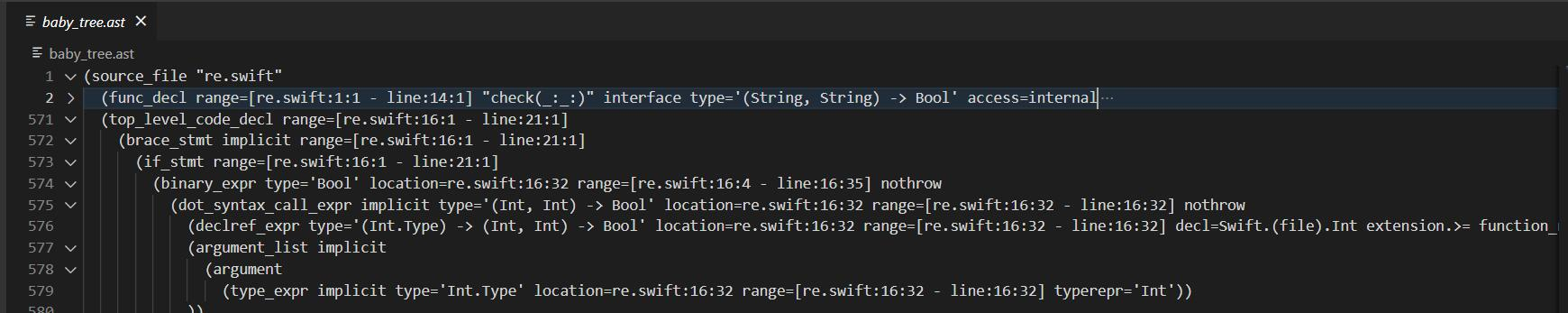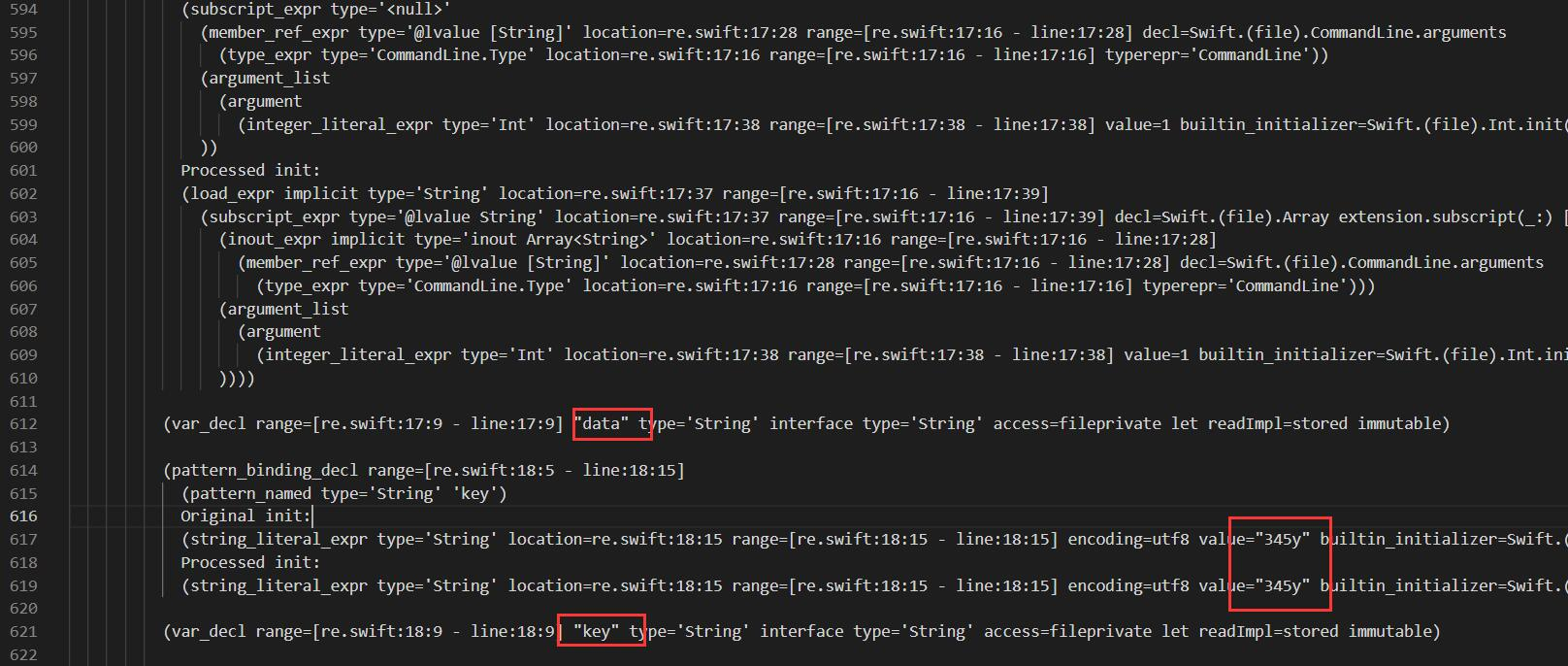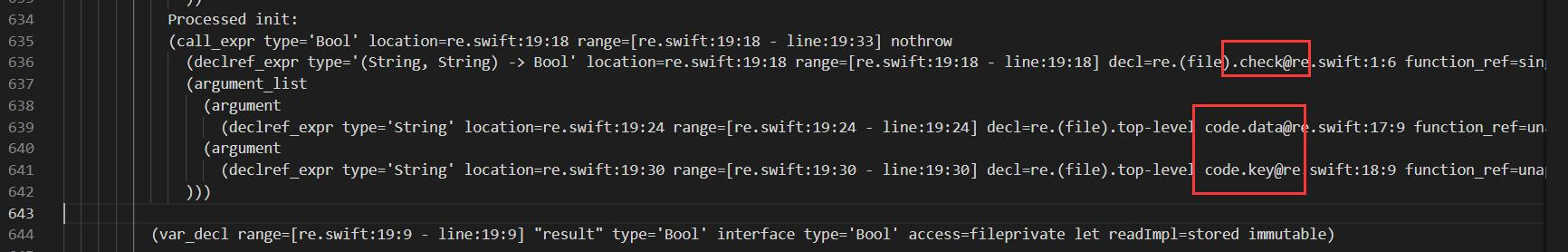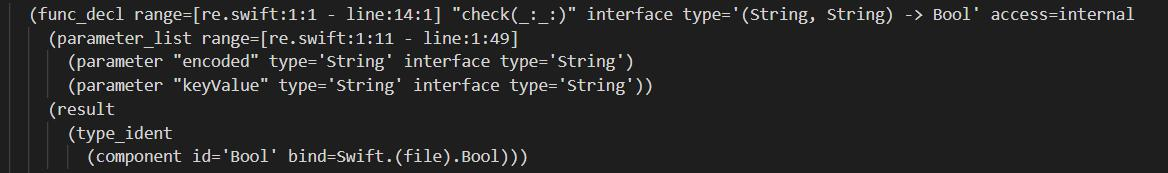for_each_stmt的直接子节点表明迭代变量是i，然后有一个直接子节点binary_expr，其操作是CloseRanged，也就是闭区间，它的argument_list带有两个参数，分别是0和一个binary_expr。这个binary_expr可以看到其操作是 Swift.(file).Int extension.-，也就是减法操作。这个减法操作的argument_list同样带有两个参数，分别是 * count re.(file).check(::).b* 和 4。那么我们就可以把这棵树画出来并整理得到这句话即为 for i in CloseRanged(0, count(b)-4)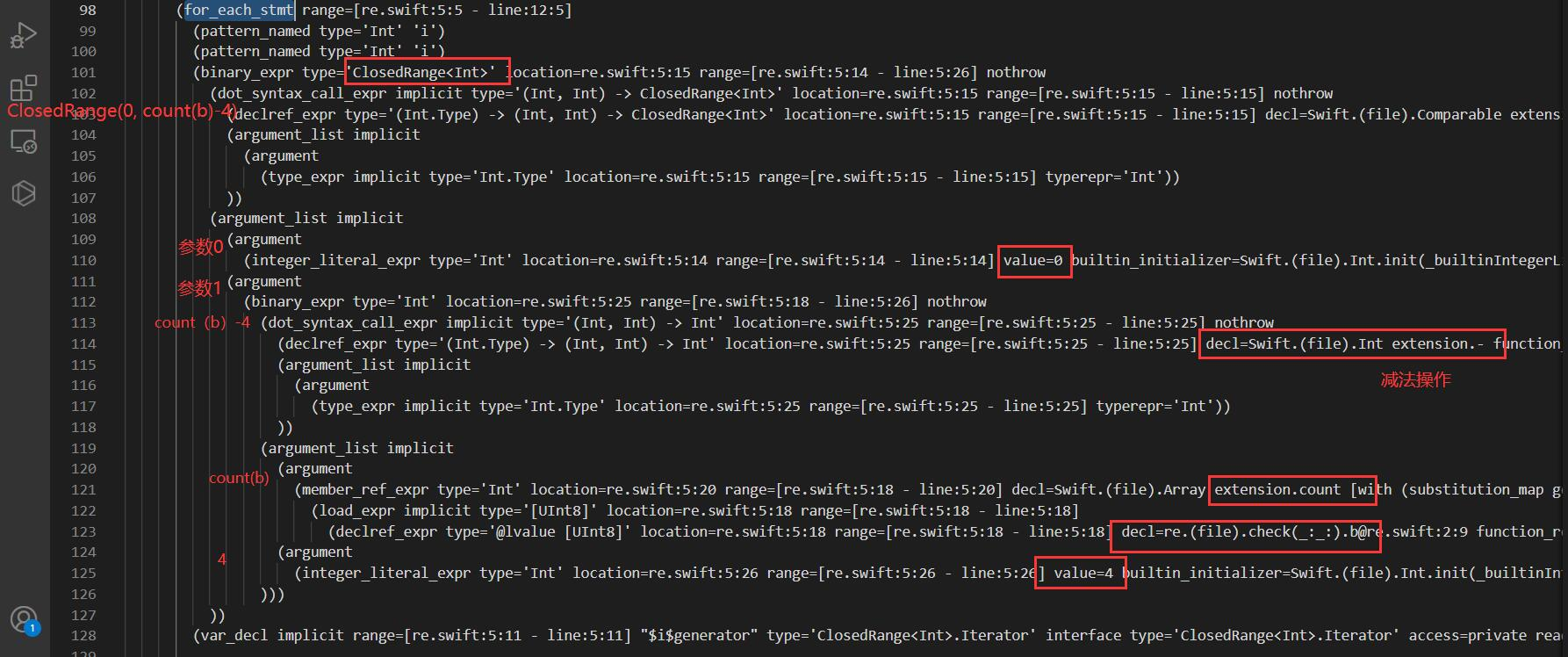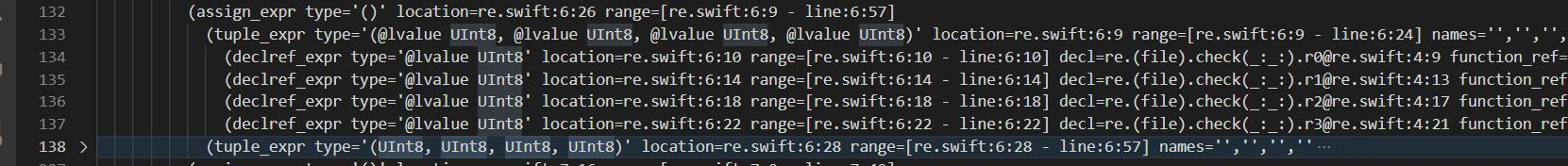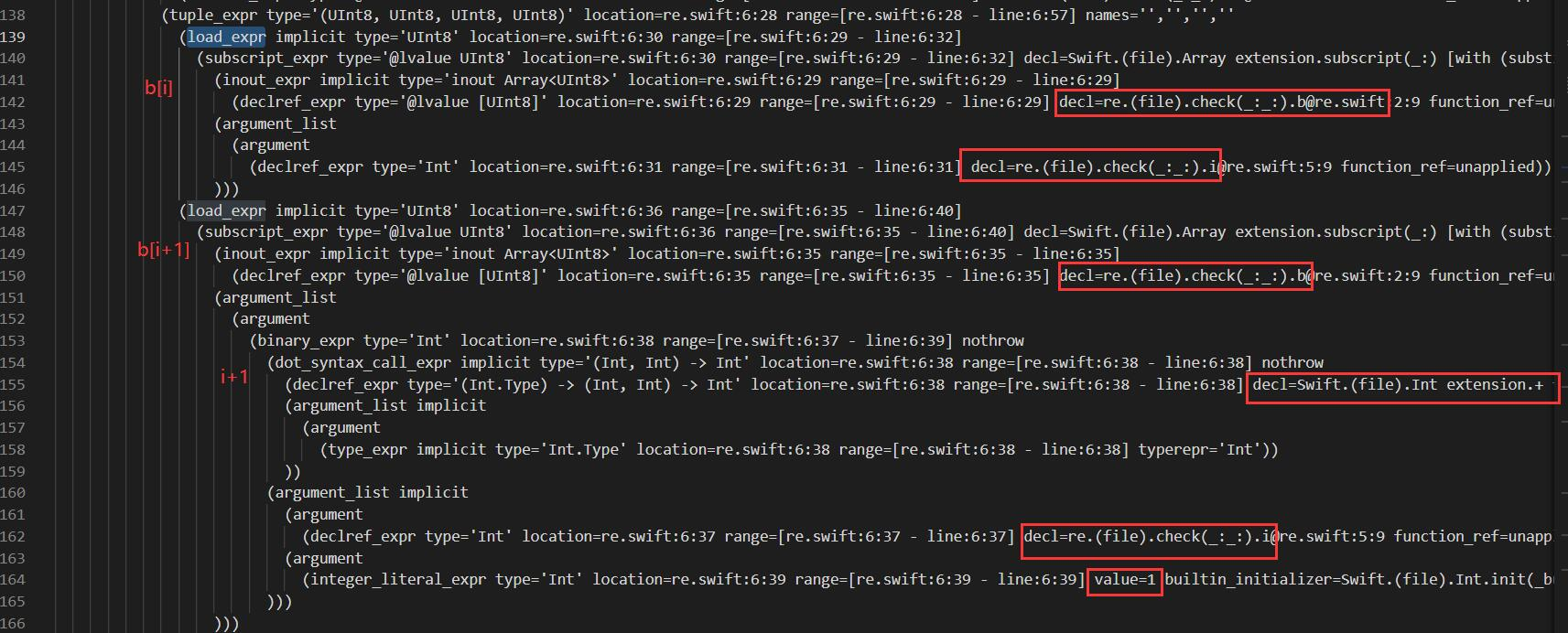### babycode

Instruction Name Operand type Semantics
OP_NOP -
OP_MOVE“ BB R(a) = R(b)
OP_LOADL” BB R(a) = Pool(b)
OP_LOADI“ BsB R(a) = mrb_int(b)
OP_LOADI_0’ B R(a) = 0
OP_LOADI_1’ B R(a) = 1
OP_LOADI_2’ B R(a) = 2
OP_LOADI_3’ B R(a) = 3
OP_LOADSYM” BB R(a) = Syms(b)
OP_LOADNIL’ B R(a) = nil
OP_LOADSELF’ B R(a) = self
OP_LOADT’ B R(a) = true
OP_LOADF’ B R(a) = false
OP_GETGV“ BB R(a) = getglobal(Syms(b))
OP_SETGV” BB setglobal(Syms(b), R(a))
OP_GETSV“ BB R(a) = Special[b]
OP_SETSV” BB Special = R(a)
OP_GETIV“ BB R(a) = ivget(Syms(b))
OP_SETIV” BB ivset(Syms(b),R(a))
OP_GETCV“ BB R(a) = cvget(Syms(b))
OP_SETCV” BB cvset(Syms(b),R(a))
OP_GETCONST“ BB R(a) = constget(Syms(b))
OP_SETCONST” BB constset(Syms(b),R(a))
OP_GETMCNST“ BB R(a) = R(a)::Syms(b)
OP_SETMCNST” BB R(a+1)::Syms(b) = R(a)
OP_GETUPVAR’ BBB R(a) = uvget(b,c)
OP_SETUPVAR’ BBB uvset(b,c,R(a))
OP_JMP S pc+=a
OP_JMPIF’ SB if R(b) pc+=a
OP_JMPNOT’ SB if !R(b) pc+=a
OP_ONERR sS rescue_push(pc+a)
OP_EXCEPT’ B R(a) = exc
OP_RESCUE“ BB R(b) = R(a).isa?(R(b))
OP_POPERR B a.times{rescue_pop()}
OP_RAISE’ B raise(R(a))
OP_EPOP B A.times{ensure_pop().call}
OP_SENDV” BB R(a) = call(R(a),Syms(b),R(a+1))
OP_SENDVB“ BB R(a) = call(R(a),Syms(b),R(a+1),&R(a+2))
OP_SEND” BBB R(a) = call(R(a),Syms(b),R(a+1),…,R(a+c))
OP_SENDB“ BBB R(a) = call(R(a),Syms(Bx),R(a+1),…,R(a+c),&R(a+c+1))
OP_CALL’ B R(a) = self.call(frame.argc, frame.argv)
OP_SUPER’ BB R(a) = super(R(a+1),… ,R(a+b+1))
OP_ARGARY’ BS R(a) = argument array (16=5:1:5:1:4)
OP_ENTER W arg setup according to flags (23=5:5:1:5:5:1:1)
OP_KARG” BB R(a) = kdict # todo
OP_KARG2“ BB R(a) = kdict; kdict.rm(Syms(b)) # todo
OP_RETURN’ B return R(a) (normal)
OP_RETURN_BLK’ B return R(a) (in-block return)
OP_BREAK’ B break R(a)
OP_BLKPUSH’ BS R(a) = block (16=5:1:5:1:4)
OP_ADD” BB R(a) = R(a)+R(a+1)
OP_SUB” BB R(a) = R(a)-R(a+1)
OP_SUBI“ BB R(a) = R(a)-C
OP_MUL” BB R(a) = R(a)R(a+1)
OP_DIV“ BB R(a) = R(a)/R(a+1)
OP_EQ” BB R(a) = R(a)==R(a+1)
OP_LT“ BB R(a) = R(a)<R(a+1)
OP_LE” BB R(a) = R(a)<=R(a+1)
OP_GT“ BB R(a) = R
OP_GE” BB R(a) = R(a)>=R(a+1)
OP_ARRAY’ BB R(a) = ary_new(R(a),R(a+1)…R(a+b))
OP_ARRAY2“ BB R
OP_ARYCAT’ B ary_cat(R(a),R(a+1))
OP_ARYPUSH’ B ary_push(R(a),R(a+1))
OP_AREF’ BB R(a) = R(a)
OP_ASET’ BB R(a) = R(a+1)
OP_APOST’ BB R(a),R(A+1)…R(A+C) = R(a)[B…]
OP_STRING” BB R(a) = str_dup(Lit(b))
OP_STRCAT’ B str_cat(R(a),R(a+1))
OP_HASH’ BB R(a) = hash_new(R(a),R(a+1)…R(a+b))
OP_HASHADD’ BB R(a) = hash_push(R(a),R(a+1)…R(a+b))
OP_LAMBDA“ BB R(a) = lambda(SEQ,OP_L_LAMBDA)
OP_BLOCK” BB R(a) = lambda(SEQ,OP_L_BLOCK)
OP_METHOD“ BB R(a) = lambda(SEQ,OP_L_METHOD)
OP_RANGE_INC’ B R(a) = range_new(R(a),R(a+1),FALSE)
OP_RANGE_EXC’ B R(a) = range_new(R(a),R(a+1),TRUE)
OP_OCLASS’ B R(a) = ::Object
OP_CLASS” BB R(a) = newclass(R(a),Syms(b),R(a+1))
OP_MODULE“ BB R(a) = newmodule(R(a),Syms(b))
OP_EXEC” BB R(a) = blockexec(R(a),SEQ)
OP_DEF“ BB R(a).newmethod(Syms(b),R(a+1))
OP_ALIAS’ B alias_method(R(a),R(a+1),R(a+2))
OP_UNDEF” BB undef_method(R(a),Syms(b))
OP_SCLASS’ B R(a) = R(a).singleton_class
OP_TCLASS’ B R(a) = target_class
OP_ERR’ B raise(RuntimeError, Lit(Bx))
OP_EXT1 - make 1st operand 16bit
OP_EXT2 - make 2nd operand 16bit
OP_EXT3 - make 1st and 2nd operands 16bit
OP_STOP - stop VM

enc_one函数接收两个四字节数和四个四字节的key做加密。加密算法是魔改的XTEA（魔改的地方有亿点多）。并且，enc_one只执行算法的一次循环，通过times控制循环轮次（轮数为YY轮，也就是16轮），通过GETUPVAR和SETUPVAR指令获取并修改上层变量来控制XTEA的v与sum（类似于lambda的变量闭包访问）。还原得到这部分加密逻辑

## 可信计算

### 基于挑战码的双向认证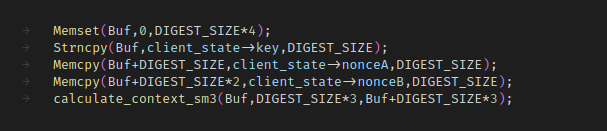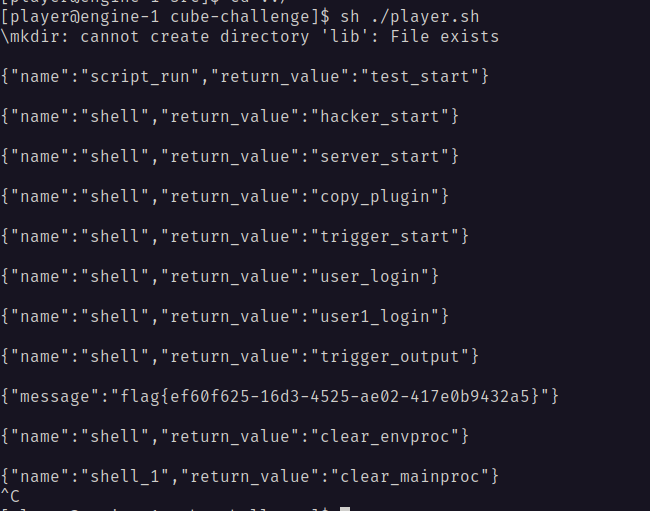### 基于挑战码的双向认证2

term计算MA=SM3(key,rB),并将MA发送给Server

### 基于挑战码的双向认证3浙ICP备19044916号-1